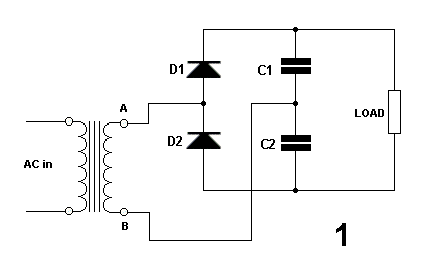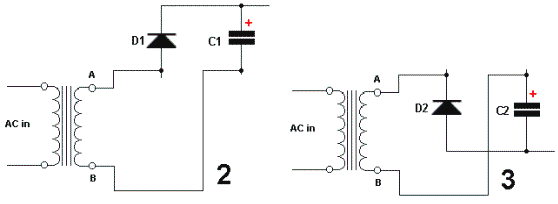Electronic Circuits and Tutorials
Tutorials
Dictionaries
Projects
Discover
Home > Electronics Tutorial > The Diode Tutorial > Voltage Doubler Tutorial
The Diode - Electronic Tutorials

# Voltage Doubler TutorialIt's best to read the page on the half-wave rectifier, first.

Point A is doing the opposite of point B.

As A increases in a positive direction, B increases negatively, and vice-versa.When A is positive, D1 is forward biased and charges C1 to the peak voltage, as in diagram 2.

D 2 is reverse biased and does not conduct.

When A goes negative, D1 is reverse biased and does not conduct.

D2 is forward biased and charges C2 to the peak voltage, as in diagram 3.

We now have two capacitors in series, each charged to the peak voltage.

The voltage across the load is. therefore, twice the peak voltage.

The voltage has been doubled.